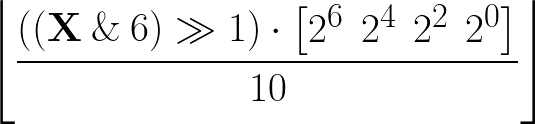## On the Subject of Unown Cipher

LEAVE NOW

This module has 5 Unown letters you can change using the up and down arrows, 4 stat values at the bottom, and a “SUBMIT” button at the top. To solve the module, submit the correct sequence of 5 Unown letters. If an incorrect sequence is entered, a strike will be recorded.

Clicking on an Unown letter shows 4 stats at the bottom. Use these numbers to decrypt the letter. Consider the 5×4 matrix X whose ith row is the vector of the four stats for the ith Unown symbol. Then, compute the following expression:This will compute a row vector of integers. Convert each to a letter based on its position in the alphabet (A=0, B=1, ..., Z=25) to determine the solution letters.

#### List of Unown Letters:

A H O V A H O V B I P W C J Q X D K R Y E L S Z F M T ? G N U !